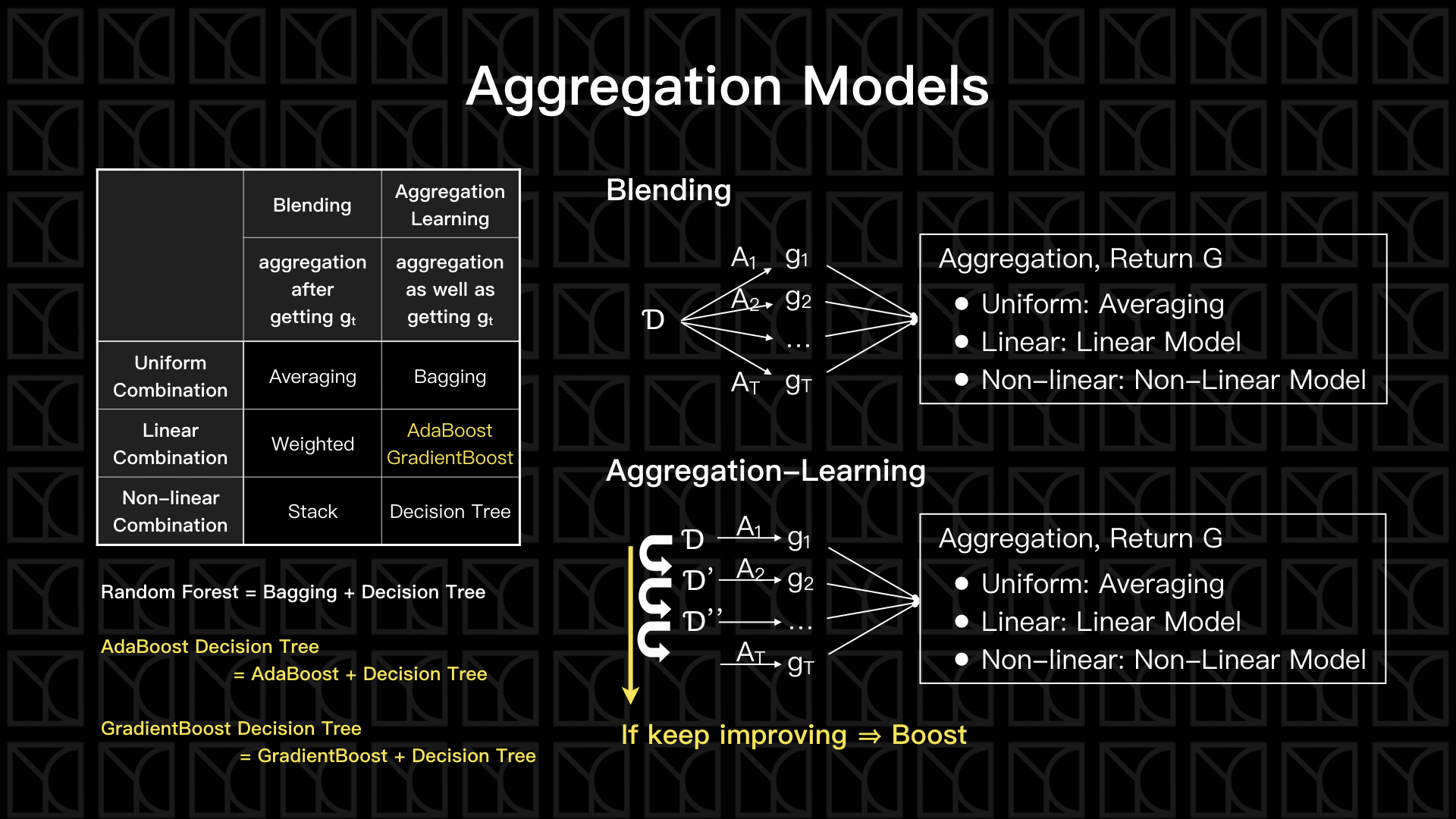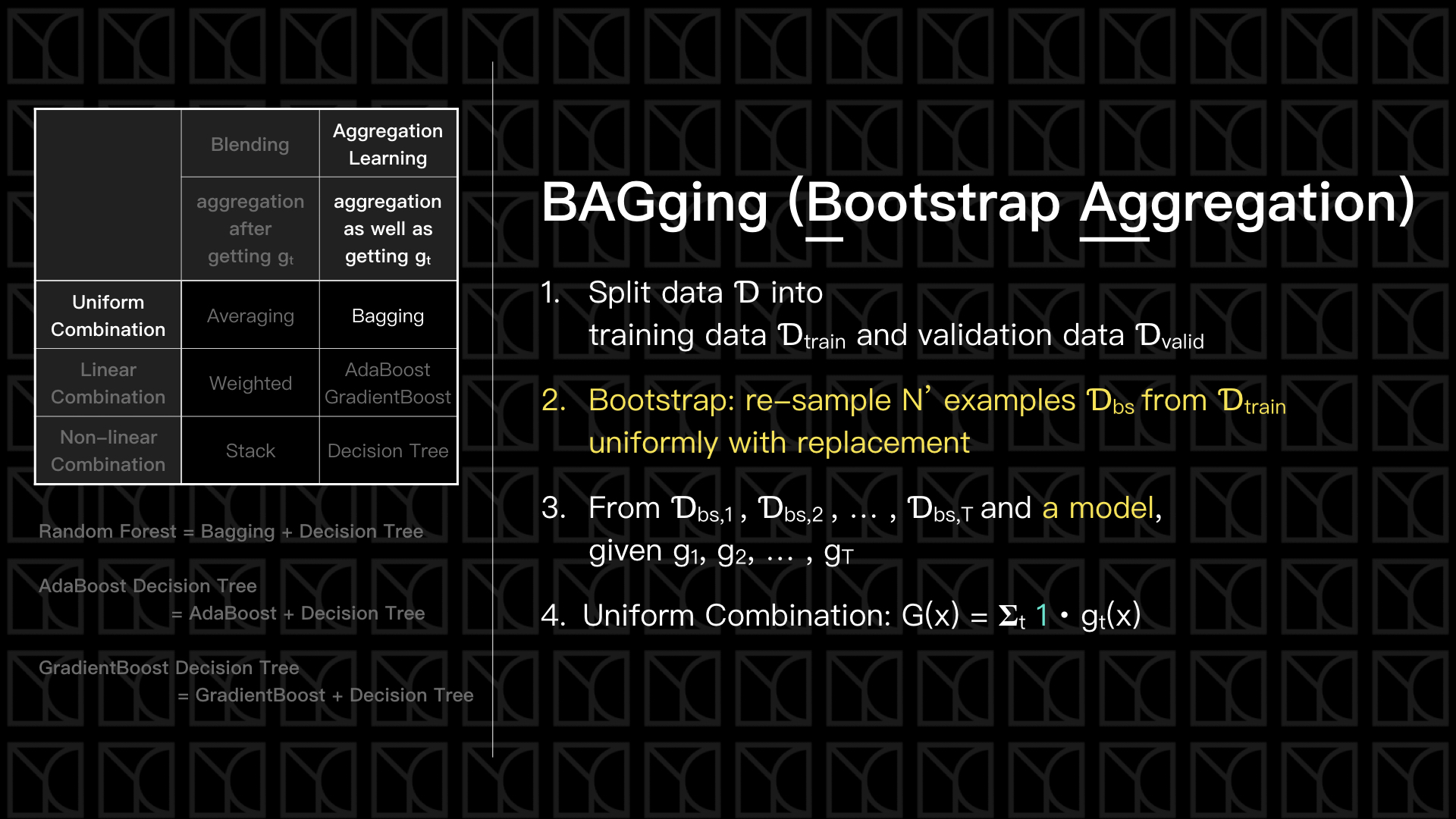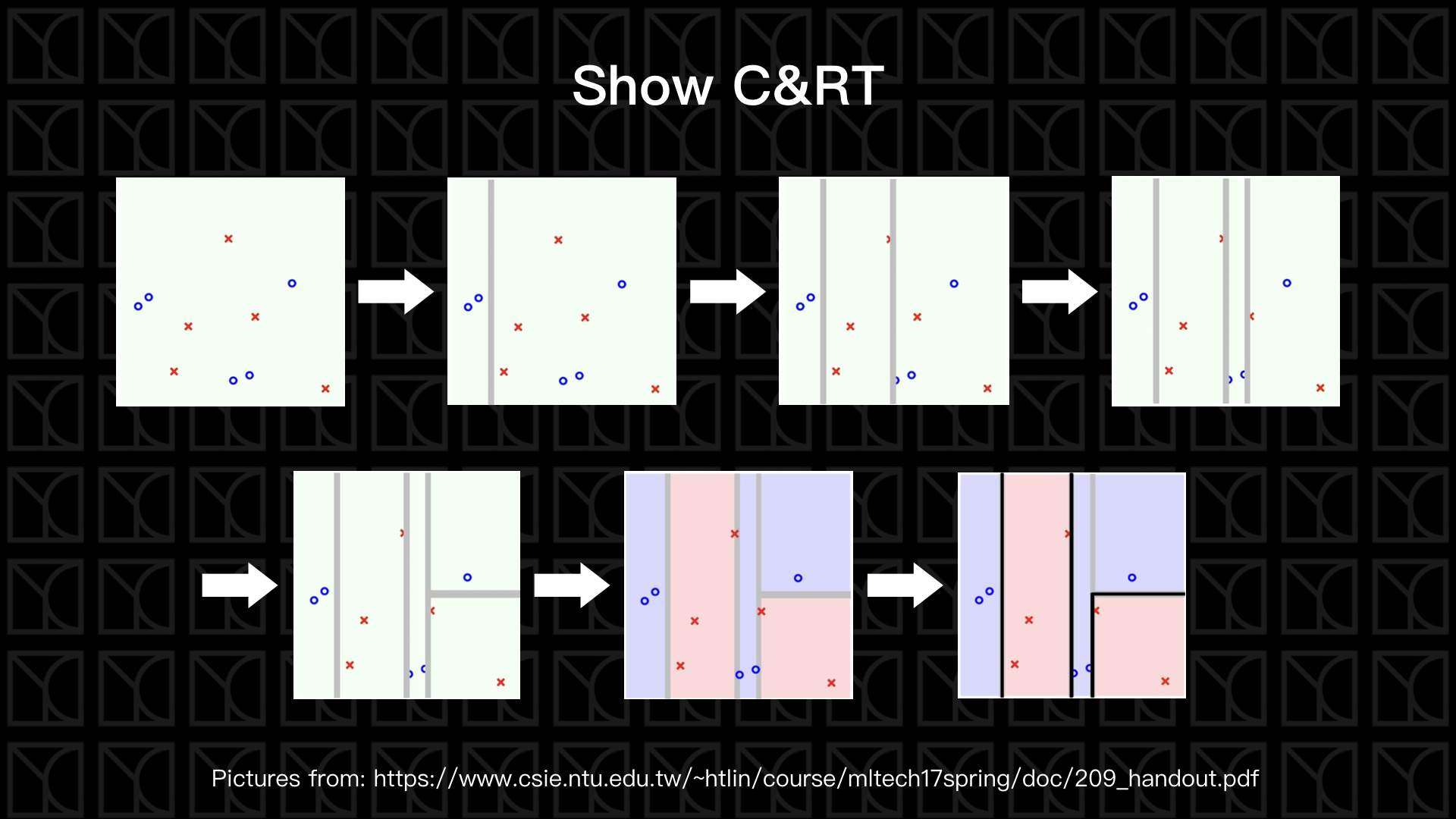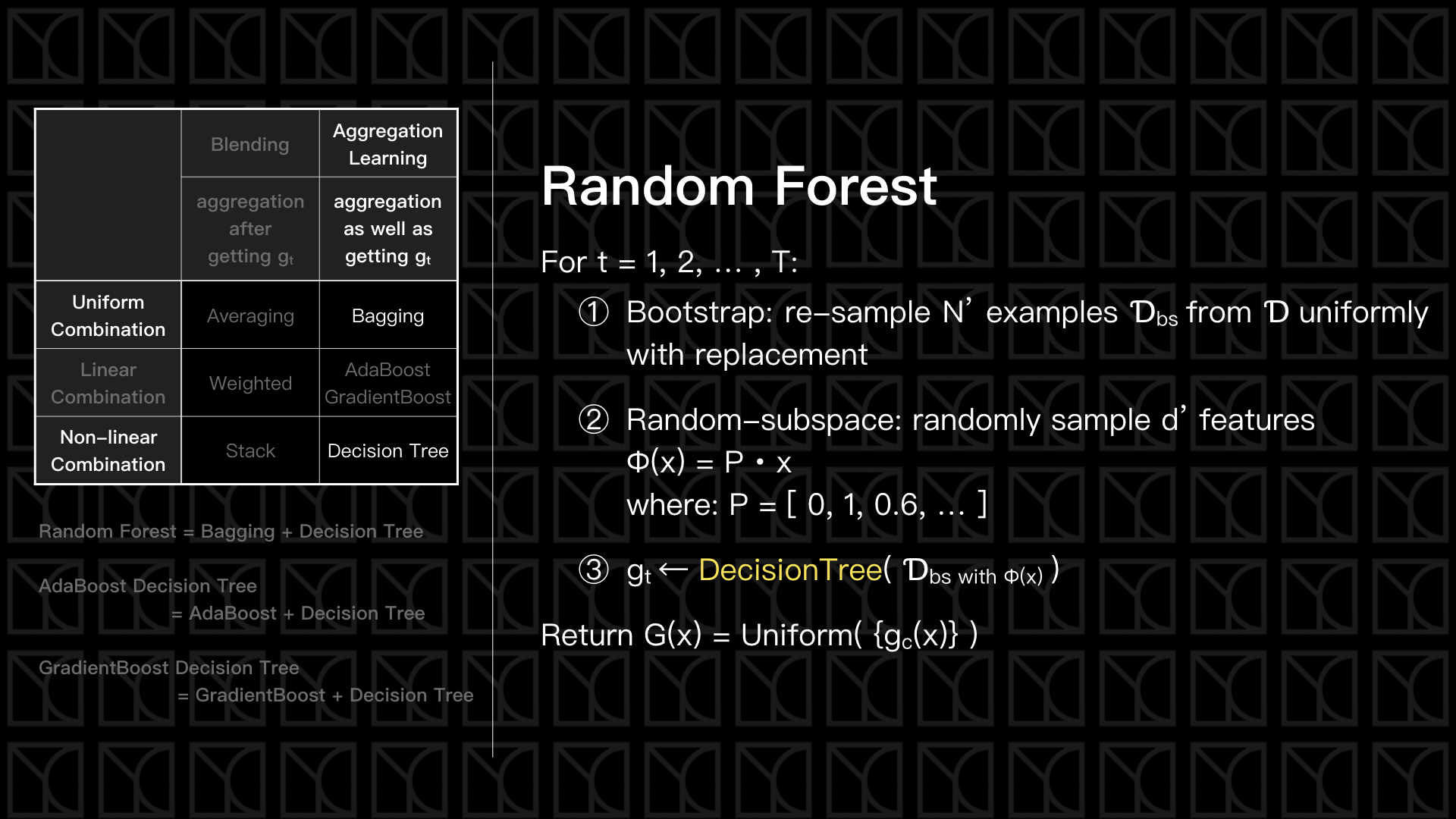# 機器學習技法 學習筆記 (4)：Basic Aggregation Models

### 綜觀Aggregation ModelsAggregation Models可以分成兩大類，第一種的作法比較簡單，先Train出一個一個獨立的Predictive Features，然後在綜合它們，「集合」的動作是發生在得到Train好的Predictive Feature之後，這叫做「Blending Models」；第二種作法則是，「集合」的動作和Training同步進行，這叫做「Aggregation-Learning Models」，Aggregation-Learning Models有一個特殊的例子叫做Boost，翻開字典查Boost的意思是「促進」，在這邊的意義是假設在Training過程所產生的Predictive Feature朝著改善Model的方向前進就叫做Boost

### Blending

Blending是泛指在Training結束之後得到幾個Predictive Features，然後再對這些Predictive Features做集合的方法### BaggingBagging是一種利用變換原本Data來造出不同$$g_{t}$$的簡單方法，Bagging的全名稱為Bootstrap Aggregation，其中Bootstrap指的是「重新取樣原有Data產生新的Data，取樣的過程是均勻且可以重複取樣的」，使用Bootstrap我們就可以從一組Data中生出多組Dataset，然後就可以使用這些Dataset來產生多組$$g_{t}$$，最後再Uniform Combination這些$$g_{t}$$，就完成了Bagging。

### Decision Tree（決策樹）1. 數據$$Ɗ$$只剩一筆數據。
2. 這群數據$$Ɗ$$已經最佳化了，我們會說它的Impurity=0，這個時候我們不知道要從哪裡再切一刀。
3. 這群數據$$Ɗ$$的Feature $$X_{n}$$都完全相同。

• $$Impurity(Ɗ) = (1/N) 𝚺_{n} ⟦y_{n}≠y^*⟧$$，其中$$y^*$$$$Ɗ$$中佔多數的類別，這個衡量方法就直接的去數出錯誤答案的比例。
• Gini Index: $$Impurity(Ɗ) = 1 - 𝚺_{k} [ 𝚺_{n}⟦y_{n}=k⟧ / N ]^{2}$$，Gini Index是最為流行的作法，它不同於上一個作法，它是在評估所有的類別後才去計算Impurity，其中 $$k$$ 代表類別。

• $$Impurity(Ɗ) = (1/N) 𝚺_{n} ( y_{n} - \overline{y} )^{2}$$，其中$$ȳ$$代表的是$$\{y_{n}\}$$的平均值，式子中使用平方誤差來評估資料的離散程度。### Random Forest（隨機森林）

Decision Tree和Bagging其實是有互補的作用，Decision Tree這種演算法是「變異度」很高的，因為它不像SVM這類的演算法，會去評估與Data之間的距離，空出最大的距離來避免Overfitting，而Bagging正可以拿來減少「變異度」，消除雜訊，所以Random Forest會比Decision Tree更不易OverfittingRandom Forest發展出了一套獨特的Validation方法，我們知道Bootstrap的結果會造成有些Data取用而有些Data不使用，而取用的Data會拿來Training，這讓你想到什麼呢？沒錯，沒有用到的Data可以做Validation，我們可以拿那些沒有被取用的Data來評估Training的好壞，我們會稱那些沒被取用的Date叫做Out-of-Bag Data，而利用Out-of-Bag Data來Validation的Error，稱為Out-of-Bag Error，

Out-of-Bag Error $$E_{oob}=(1/N) 𝚺_{n} err(y_{n}, {G_{n}}^{-}(x_{n}))$$

$$where:\ {G_{n}}^{-}(x) = Average(Models\ without\ using\ this\ data)$$

Out-of-Bag Error提供一個很方便的Self-validation的方法。

$$important(i) = E_{oob}(G) - E_{oob}(G with random-subspace at i)$$